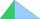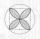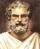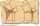# Same area

There is a given triangle. Construct a square of the same area.

Result

x=##:  0

Leave us a comment of example and its solution (i.e. if it is still somewhat unclear...):

Showing 0 comments:Be the first to comment!#### To solve this example are needed these knowledge from mathematics:

See also our right triangle calculator. See also our trigonometric triangle calculator.

## Next similar examples:

1. Right angledFrom the right triangle with legs 12 cm and 20 cm we built a square with the same content as the triangle. How long will be side of the square?
2. Right 24Right isosceles triangle has an altitude x drawn from the right angle to the hypotenuse dividing it into 2 unequal segments. The length of one segment is 5 cm. What is the area of the triangle? Thank you.
3. QuatrefoilCalculate area of the quatrefoil which is inscribed in a square with side 6 cm.
4. Circle annulusThere are 2 concentric circles in the figure. Chord of larger circle 10 cm long is tangent to the smaller circle. What are does annulus have?
5. Circle tangentIt is given to a circle with the center S and radius 3.5 cm. Distance from the center to line p is 6 cm. Construct a circle tangent n which is perpendicular to the line p.
6. Euclid2In right triangle ABC with right angle at C is given side a=27 and height v=12. Calculate the perimeter of the triangle.
7. Square and circlesSquare with sides 61 mm is circumscribed and inscribed with circles. Determine the radiuses of both circles.
8. Triangle KLMIn the rectangular triangle KLM, where is hypotenuse m (sketch it!) find the length of the leg k and the height of triangle h if hypotenuse's segments are known mk = 5cm and ml = 15cm
9. SemicircleIn the semicircle with center S and the diameter AB is constructed equilateral triangle SBC. What is the magnitude of the angle ∠SAC?
10. Triangle ABCIn a triangle ABC with the side BC of length 2 cm The middle point of AB. Points L and M split AC side into three equal lines. KLM is isosceles triangle with a right angle at the point K. Determine the lengths of the sides AB, AC triangle ABC.
11. Isosceles IVIn an isosceles triangle ABC is |AC| = |BC| = 13 and |AB| = 10. Calculate the radius of the inscribed (r) and described (R) circle.
12. Euclid 5Calculate the length of remain sides of a right triangle ABC if a = 7 cm and height vc = 5 cm.
13. Triangle P2Can triangle have two right angles?
14. Euclid3Calculate height and sides of the right triangle, if one leg is a = 81 cm and section of hypotenuse adjacent to the second leg cb = 39 cm.
15. RT and circlesSolve right triangle if the radius of inscribed circle is r=9 and radius of circumscribed circle is R=23.
16. Chord - TS v2The radius of circle k measures 87 cm. Chord GH = 22 cm. What is TS?
17. Theorem proveWe want to prove the sentense: If the natural number n is divisible by six, then n is divisible by three. From what assumption we started?